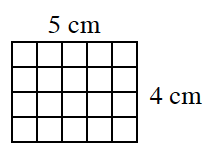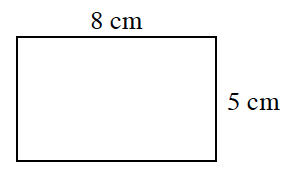### Home > MC2 > Chapter 1 > Lesson 1.1.2 > Problem1-12

1-12.

In this lesson, you looked at the number of tiles and number of toothpicks used to form shapes made of square tiles as you played the "Toothpick and Tiles" game. The math words that also describe the number of tiles and toothpicks are area and perimeter. Read the Math Notes box for this lesson to review how area and perimeter are related to tiles and toothpicks, then answer the questions below.

1. Find the area and perimeter of the figure at right.

The area is the number of square units in the shape. The perimeter is the total length of all the sides.

Add up all the sides of the rectangle: $5\text{ cm} + 5\text{ cm} + 4\text{ cm} + 4\text{ cm}$

Count the squares in the rectangle. How does the number of squares relate to the base and height?1. Find the area and perimeter of the rectangle at right.

See part (a).

Area: $40$ sq cm
Perimeter: $26$ cm
Be sure to explain how these answers relate to the given
measurements of the rectangle.1. Now design your own shape with $5$ square tiles. Record the perimeter and the area. (CPM).

Area: $5$ sq in
Perimeter: $12$ in

Use the eTool below to design a shape with 5 square tiles for part (c).
Click the link at right for the full version of the eTool: MC2 1-12 HW eTool